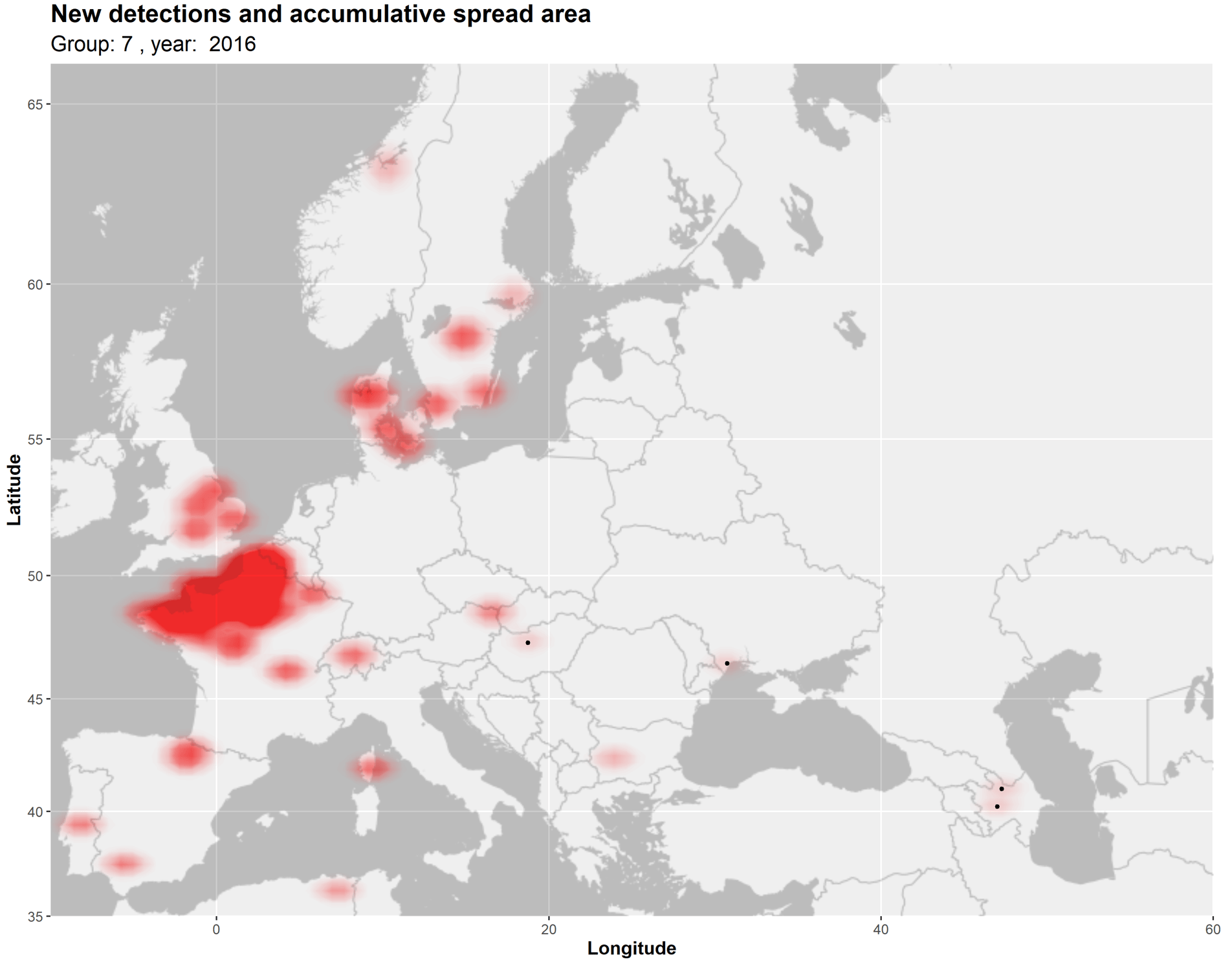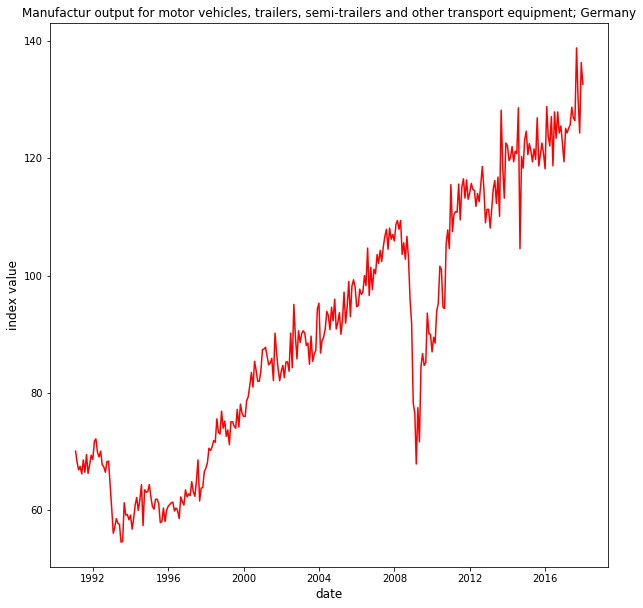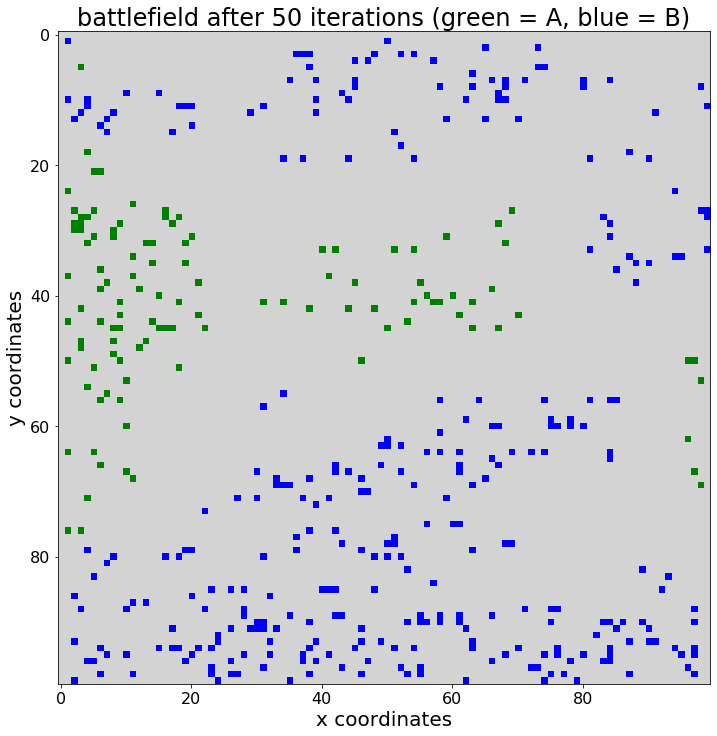# Python programming

## Integer programming for manufacturing implemented with CPLEX & DOCPLEXThanks to the evolution of Python and its applications to solve linear programs and their variations supply chain and operations research analysts now have access to numerous packages and tools that support decision making. In this article I will use […]In this blog post I will discuss one of the basic types of linked lists i.e. singly-linked lists. This is one of the important and simple data structure types. Furthermore, this is a data structure that can be defined by […]

## Spatial data visualization pipeline in Python and RThis article introduces the concept of spatial data visualization pipeline in R or Python. I have written various posts covering spatial data visualization for supply chain analysis and management, in both R and Python. Relevant libraries comprise e.g. Leaflet in […]

## Spatial heatmaps in Python using Leaflet.js via Folium moduleIn previous posts I have demonstrated how one can geocode data and plot markers using Geopy and Folium in Python. I have also demonstrated heatmapping workflows in R, using packages such as Leaflet. In this post I want to use […]

## Multiple geocoded map markers with Geopy and Folium in PythonIn a previous post I have already demonstrated how to use Nominatim in Python (using the Geopy module) to geocode a location name into longitude and latitude coordinates. In this post I want to show how one can geocode a […]

## Intro to map-based visualization in Python, using FoliumUsing Folium in Python one can display maps. Folium can be installed using pip install. Folium make use of the leaflet.js library. In a previous post I already demonstrated how to e.g. plot markers and heatmaps onto maps in R, […]

## Developing an agent-based framework for simulation models in PythonIn a previous post I constructed a simple agent-based simulation model, containing groups of agents that can be located on a battlefield grid. The model was coded in Python, using matplotlib for visualization. I went on to conduct a simple […]

## String operations in PythonThis is a quick documentation on Python string operations. Writing string to variable (assignment) using equal (=) operator a = “test string” print(a) test string Line-breaks within a string (multi-line string) using three quotes b = “””This is first line, […]

## Retrieving platinum and palladium prices from Quandl in PythonIn a previous post I demonstrated how one can query automotive data via Quandl directly from within a Python script. In this post I will document how to query platinum and palladium exchange prices from Quandl in Python. The data […]

## Querying automotive industry data from Quandl in PythonA quick post on how to query data from Quandl in Python. Quandl can be installed with the “pip install” command in your command prompt. For this simply type “pip install quandl” in your Anaconda command prompt. Quandl is the […]

## Calculating coefficients for the first derivative of a continuous function (Python)A quick exercise in implementing an algorithm calculates the coefficients of the first derivative of a continuous function with one variable. Implementation is done in the code below. The function takes a list of coefficients. These must be specified in […]

## Algorithm for finding maximum value in list (Python)As a small coding exercise I will write some lines of Python code that find the maximum value in a list and return it. In Python, this can be done with a build-in default function called max(). I avoid using […]

## Algorithm for finding smallest value in list (Python)As a small coding exercise I will write some lines of Python code that find the minimum value in a list and return it. In Python, this can be done with a build-in default function named min(). I avoid using […]

## A simple agent-based simulation run, visualized using Matplotlib in PythonIn a previous post I constructed a simple agent-based simulation model, containing groups of agents that can be located on a battlefield grid. The model was coded in Python, using matplotlib for visualization. The agents were modelled as a class, […]

## Iterables and iterators in PythonA brief documentation on iterables and iterators in Python. An iterator is an object that can be iterated, i.e. one can traverse through all of its elements. Iterables are containers that can provide an iterator object. Examples of iterables are […]